eBlocks : Embedded Systems Building Blocks University of California, Riverside Department of Computer Science Riverside, CA 92521Home

Number eBlock Catalog

 Sensor eBlocks eBlock Diagram Description Interface Temperature Sensor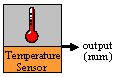Device outputs the current temperature in Fahrenheit. output = current temp (F) Distance Sensor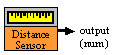Sensor outputs the distance between the device and another object. output = distance (ft)

 Output/Display eBlocks eBlock Diagram Description Interface Integer Display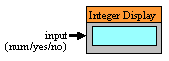Device displays the integer value recieved. Displays the input.

 Arithmetic/Logic eBlocks eBlock Diagram Description Interface Threshold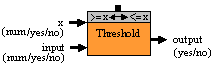Device determines if input is <= x or input >= x, depending on what switch is set to. >= x MODE: output = yes, (input >= x) output = no, (input < x) <= x MODE: output = yes, (input <= x) output = no, (input > x) Range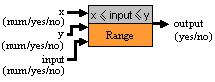Device determines if the input is within the x and y range (inclusive). output = yes, x <= input <= y output = no, input < x OR input > y Equation/Math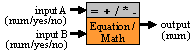User defines the function of the math block, and the block calculates A function B. output = A function B Turnstile Counter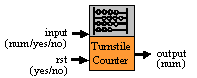The value held by the counter is incremented by the input value. The rst input will reset this value to zero. INPUT: input = yes, increment value held by 1 input = no, increment value held by 0 input = num, increment value held by num OUTPUT: output = value held by counter block Constant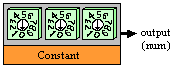The user specifies a constant value. value = user defined

 Timer eBlocks eBlock Diagram Description Interface Seconds Timer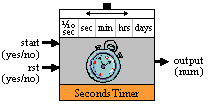The user selects the granularity of the time kept track of. When timer is activated it counts by the granularity specified. INPUT: start = yes, activate time start = no, stop nothing rst = yes, reset the timer to zero OUTPUT: output = time passed since start set to yes and rst set to no.

 Communication eBlocks eBlock Diagram Description Interface Wireless Transmitter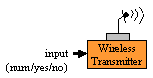Device wirelessly transmits a signal. yes = input is yes no = input is no Wireless Receiver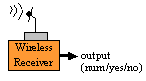Device wirelessly receives a signal. yes = yes recieved no = no recieved Splitter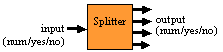Receives a signal and replicates that signal on each output. OUTPUT: yes = input yes no = input no

 Miscellaneous eBlocks eBlock Diagram Description Interface Turnstile Logger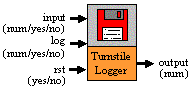Device keeps a list of inputs; the input value is logged when the log input is yes. Later the list of inputs can be retrieved from the logger unit. The list is cleared when reset is yes. *We have not yet defined how to read the times from the logger. log = yes, log the current input value log = no, do nothing rst = yes, clear input values held by logger

• weight sensor
• pressure sensor
• moisture sensor
• voice recognition
• wind sensor
• flow meter
• selective splitter(int sent to indicate which out goes to yes)
• data logger (tracks the int value instead of yes/no)
• dimmer/intensifier - most likely useful in indicating needed signal strength in rx/tx devices

eBlock Catalog
›   Yes/No eBlocks
›   Number eBlocks
Sample eBlock Systems
›  Yes/No eBlocks
›  Number eBlocks
Simulator
Publications
Gallery
People